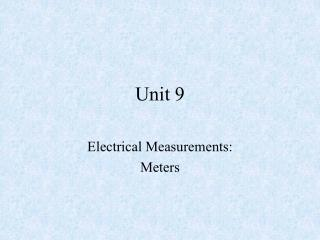DownloadDownload PresentationUnit 9

# Unit 9

Download Presentation## Unit 9

- - - - - - - - - - - - - - - - - - - - - - - - - - - E N D - - - - - - - - - - - - - - - - - - - - - - - - - - -
##### Presentation Transcript

1. Unit 9 Electrical Measurements: Meters

2. Objectives • After completing this chapter, the student should be able to: • Identify the two types of meter movements available. • Describe how a voltmeter is used in a circuit. • Describe how an ammeter is used in a circuit. • Describe how an ohmmeter is used for measuring resistance.

3. Identify the functions of a multimeter. • Identify the advantages and disadvantages of DMM’s and VOM’s. • Describe how to use a multimeter to measure voltage, current, and resistance. • Describe how to measure current using an ammeter. • Describe how to connect an ammeter into a circuit. • List safety precautions for using an ammeter.

4. Describe how to connect a voltmeter to an electrical circuit. • List safety precautions for connecting a voltmeter to a circuit. • Describe how resistance values are measured using an ohmmeter. • Define continuity check. • Describe how an ohmmeter is used to check open, short, or closed circuits.

5. METERS • Detect and measure the invisible action of electrons. • Are indispensable in examining the operation of a circuit. • Two types are available.

6. Analog meter • Graduated scale with a pointer. • Provides a better graphic display of rapid changes. • Must be adjusted to zero before use.

7. Digital meter • Provides a reading in numbers. • Easier to read. • Provides a more accurate reading.

8. Polarity of the terminals • Red is positive. • Black is negative.

9. Ammeter • Measures current in a circuit. • Can be considered a flow meter. • Measures the number of electrons flowing past a given point in a circuit. • Must be connected in series with the load • Has a very low impedance

10. Voltmeter • Measures the voltage between two points in a circuit. • Can be considered a pressure gauge.

11. Ohmmeter • Measures resistance by placing a voltage across the device to be measured, inducing a current flow through the device.

12. Multimeter • Combines the voltmeter, ammeter, and the ohmmeter into a single instrument. • An analog multimeter is also referred to as a volt-ohm-milliammeter (VOM). • A digital multimeter is also referred to as a DMM.

13. Advantages of DMM’s • Easier to read. • Auto-ranging capabilities. • Auto-zeroing for resistance reading. • Auto lock capability.

14. Disadvantages of DMM’s • Requires external power source. • Excessive voltage can damage meter. • Limitations due to the sampling rate.

15. Measuring current • Turn off the power. • Open the circuit. • Insert the ammeter into the circuit series. • When correctly connected, the needle will move from left to right. • Never connect in parallel with any circuit component.

16. Never connect an ammeter directly to a voltage source. • Set the meter to its highest scale before turning on the power.

17. Measuring voltage • Remove the power source. • Connect the voltmeter. • Pay close attention to polarity. • Set meter to its highest scale. • Reapply power.

18. Measuring resistance • Remove power supply. • Disconnect one end of the component from the circuit. • Connect the ohmmeter leads across the device.

19. Use an ohmmeter to determine whether a circuit is: • Open • Has infinite resistance because no current flows through it. • Shorted • Has zero ohms of resistance because current flows through it without developing a voltage drop.

20. Closed • A complete path for current where resistance varies depending on the components in the circuit. • Continuity check • The testing for an open, short, or closed circuit.

21. Reading meter scales • Voltmeter and ammeter scales. • Read from left to right. • Value of voltage or current is read under the pointer.

22. Ohmmeter scale • Read from right to left. • Nonlinear. • Must be calibrated before use.

23. In Summary • Meters • Analog • Digital • Ammeter • Voltmeter • Ohmmeter

24. Multimeters • VOM • DMM